Courses

# Uncontrolled Intersection - 3 Civil Engineering (CE) Notes | EduRev

## Civil Engineering (CE) : Uncontrolled Intersection - 3 Civil Engineering (CE) Notes | EduRev

The document Uncontrolled Intersection - 3 Civil Engineering (CE) Notes | EduRev is a part of Civil Engineering (CE) category.
All you need of Civil Engineering (CE) at this link: Civil Engineering (CE)

Movement capacity and impedance effects

Vehicles use gaps at a TWSC intersection in a prioritized manner. When traffic becomes congested in a high-priority movement, it can impede lower-priority movements that are streams of Ranks 3 and 4 as shown in Fig. 30:4 from using gaps in the traffic stream, reducing the potential capacity of these movements. The ideal potential capacities must be adjusted to reflect the impedance effects of higher priority movements that may utilize some of the gaps sought by lower priority movements. This impedance may come due to both pedestrians and vehicular sources called movement capacity.

The movement capacity is found by multiplying the potential capacity by an adjustment factor. The adjustment factor is the product of the probability that each impeding movement will be blocking a subject vehicle. That is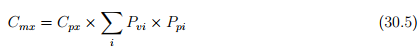where, Cmx is the movement capacity in vph, Cpx is the potential capacity movement x in vph, Pvi is the probability that impeding vehicular movement i is not blocking the subject flow; (also referred to as the vehicular impedance factor for movement i, Ppi is the probability that impeding pedestrian movement j is not blocking the subject flow; also referred to us the pedestrian impedance factor for the movement j.

Vehicular movements

Priority 2 vehicular movements LTs from major street and RTs from minor street are not impeded by any other vehicular flow, as they represent the highest priority movements seeking gaps. They are impeded, however, by Rank 1 pedestrian movements. Priority 3 vehicular movements are impeded by Priority 2 vehicular movements and Priority l and 2 pedestrian movements seeking to use the same gaps. Priority 4 vehicular movements are impeded by Priority 2 and 3 vehicular movements, and Priority 1 and 2 pedestrian movements using the same gaps. Table. 30:3 lists the impeding flows for each subject movement in a four leg. Generally the rule stated the probability that impeding vehicular movement i is not blocking the subject movement is computed as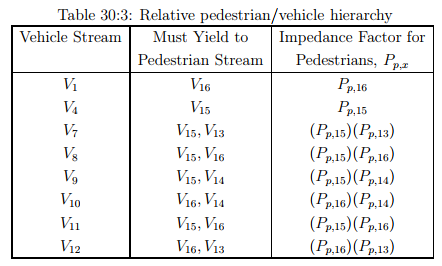where, vi is the demand flow for impeding movement i, and Cmi is the movement capacity for impeding movement i vph. Pedestrian impedance factors are computed as:

Pedestrian Movements

One of the impeding effects for all the movement is pedestrians movement. Both approaches of Minor-street vehicle streams must yield to pedestrian streams. Table. 30:3 shows that relative hierarchy between pedestrian and vehicular streams used. A factor accounting for pedestrian blockage is computed by Eqn. 30.7 on the basis of pedestrian volume, the pedestrian walking speed, and the lane width that is: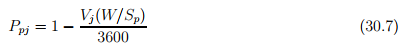where, ppj is the pedestrian impedance factor for impeding pedestrian movement j, vj is the pedestrian flow rate, impeding movement j in peds/hr, w is the lane width in m, and Sp is the pedestrian walking speed in m/s.

Determining Shared Lane Capacity

The capacities of individual streams (left turn, through and right turn) are calculated separately. If the streams share a common traffic lane, the capacity of the shared lane is then calculated according to the shared lane procedure. But movement capacities still represent an assumption that each minor street movement operates out of an exclusive lane. Where two or three movements share a lane its combined capacity computed as: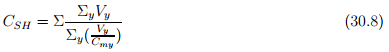where, CSH is the shared lane capacity in veh/hr, Vis the flow rate, movement y sharing lane with other minor street flow, and Cmy is the movement capacity of movement y sharing lane with other minor street.

Determining control delay

Delay is a complex measure and depends on a number of variables it is a measure of driver discomfort, frustration, fuel consumption, increased travel time etc. Total delay is the difference between the travel time actually experienced and the reference travel time that would result during base conditions, in the absence of incident, control, traffic, or geometric delay. Also, Average control delay for any particular minor movement is a function of the Capacity of the approach and The degree of saturation. The control delay per vehicle for a movement in a separate lane is given by: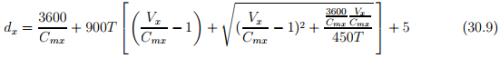where, dx is the average control delay per vehicle for movement x in s/veh, Cmx is the capacity of movement or shared lane x in veh/hr, T is the analysis period h (15 min=0.25 h), and Vx is the demand flow rate, movement or shared lane x in veh/hr.

Performance measures

Four measures are used to describe the performance of TWSC intersections: control delay, delay to major street through vehicles, queue length, and v/c ratio. The primary measure that is used to provide an estimate of LOS is control delay. This measure can be estimated for any movement on the minor (i.e., the stop-controlled) street. By summing delay estimates for individual movements, a delay estimate for each minor street movement and minor street approach can be achieved.

For AWSC intersections, the average control delay (in seconds per vehicle) is used as the primary measure of performance. Control delay is the increased time of travel for a vehicle approaching and passing through an AWSC intersection, compared with a free flow vehicle if it were not required to slow or stop at the intersection. According to the performance measure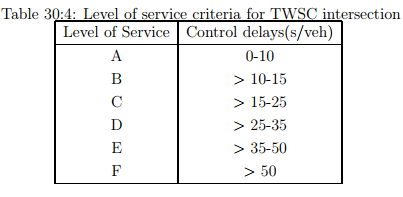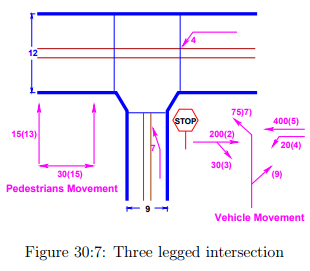of the TWSC intersection, LOS of the minor-street left turn operates at level of service C approaches to B.

Numerical example

For a three legged intersection given in figure 30:7 determine the control delay and level of service for movement 7. The total volume of both pedestrian and vehicular traffic at each movement is given in the figure itself. Following data is also given:

• The speed of the pedestrians is 1.2m/s

• All flows contains 10% trucks

• The percentage of the grade is 0.00

• Ignore moments coming from south bound

• The analysis period is 15 min. (T=0.25)

Solution:

1. Compute the critical gap and follow up time:

(a) Critical gap tcx = tcb + tcHV PHV + tcGGtcT tLT . From table. 30:1 and table. 30:2 we have tcb = 7.1 s , tcG = 0.2, tcT = 0.0, tLT = 0.0. Then tcx at movement 7 computed as: tc7 = 7.1 + 1.0 × 0.1+0.2 × 0.0 - 0.0 - 0.0 = 6.50 sec

(b) To compute the Follow up time: From table. 30:1 and table. 30:2 we have tfb = 3.5 s , tfHV = 0.9. Then tfx at movement 7 computed as: tfx = tfb + tfHV PHV tf7 = 3.5 + 0.9 × 0.1 = 3.59 sec.

2. Compute the conflicting flow rate:

Vc7 = 2V4 + V5 + V13 + V2 + 0.5V3 + V15
= 40 + 400 + 15 + 200 + 0.5 × 30 + 30
= 700 conflicts/hr

3. Determining potential capacity: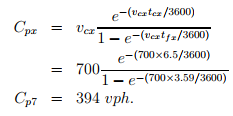4.Determine the impudence effect of the movement capacity for movement 7: From the given figure movement 7 is impeded by vehicular movement 4 and 1 and pedestrian 13 and 15. (a) Pedestrian impedance probability computed as: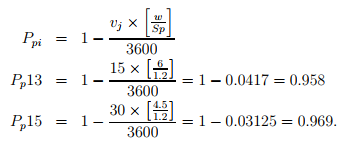(b) Vehicular impedance probabilities are: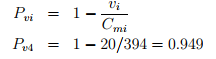(c) Once the pedestrian and vehicular impedance is determined, the moment capacity is computed as: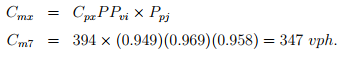5. Delay computation: The delay is Calculated by using the formula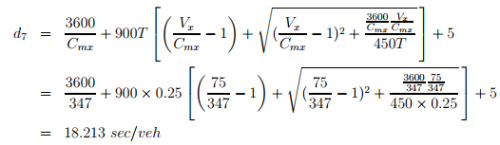The delay of movement 7 is 18.213 sec/veh.

6. Determine the level of service: From the computed delay (18.213 se) in step 5 the level of service is LOS C obtained from HCM table.

Conclusion

This chapter focuses on theoretical analysis of capacity at uncontrolled intersections. First the gap acceptance theory and follow time was described; including conflict volume determination through the hierarchy of priorities for two ways stop controlled intersection. Second, after determining the potential capacity using the computed value and then prepare an adjustment for this capacity. Finally, computation of the delay to determine the level of service (LOS) of the given intersection is also described.

Offer running on EduRev: Apply code STAYHOME200 to get INR 200 off on our premium plan EduRev Infinity!

,

,

,

,

,

,

,

,

,

,

,

,

,

,

,

,

,

,

,

,

,

;